Courses

# Ex 8.4 NCERT Solutions- Introduction to Trigonometry Class 10 Notes | EduRev

## Class 10 : Ex 8.4 NCERT Solutions- Introduction to Trigonometry Class 10 Notes | EduRev

The document Ex 8.4 NCERT Solutions- Introduction to Trigonometry Class 10 Notes | EduRev is a part of the Class 10 Course Mathematics (Maths) Class 10.
All you need of Class 10 at this link: Class 10

Q.1. Express the trigonometric ratios sin A, sec A and tan A in terms of cot A.
Sol.  From trigonometric identity,
cosec2 A - cot2 A = 1, we get
cosec2 A = 1 + cot2A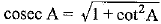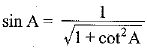Again from trigonometric identity
sec2 A - tan2 A = 1
⇒ sec2 A = 1+ tan2 A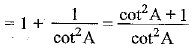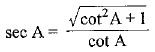Since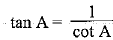Q.2. Write all the other trigonometric ratios of ∠A in terms of sec A.
Sol. Since sin2 A+ cos2 A = 1,
therefore sin2 A = 1 - cos2A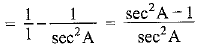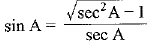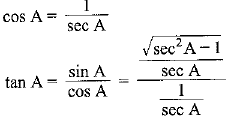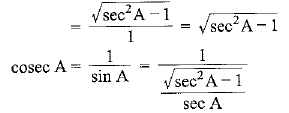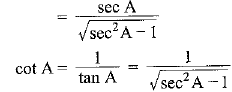Q.3. Evaluate: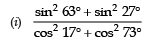(ii) sin 25° cos 65° + cos 25° sin 65°
Sol.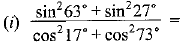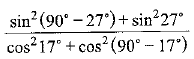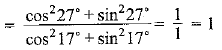(ii) sin 25° cos 65° + cos 25° sin 65°
= sin 25° cos (90° - 25°) + cos 25° sin (90° -25°)
= sin 25° sin 25° + cos 25° cos 25°
= sin2 25° + cos2 25° = 1

Q.4. Choose the correct option. Justify your choice.
(i) 9 sec2 A − 9 tan2 A =
(A) 1
(B) 9
(C) 8
(D) 0
(ii) (1 + tan θ + sec θ) (1 + cot θ − cosec θ) =
(A) 0

(B) 1
(C) 2
(D) − 1
(iii) (sec A + tan A) (1 − sin A) =
(A) sec A
(B) sin A
(C) cosec A
(D) cos A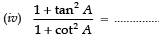(A) sec2 A
(B) −1
(C) cot2 A
(D) tan2 A

Sol. (i) Since, 9 sec2 A − 9 tan2 A = 9 (sec2 A − tan2 A)
= 9 x 1 = 9
∴ The option (B) is correct.
(ii)  (1 + tan θ + sec θ) (1 + cot θ − cosec θ)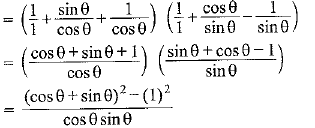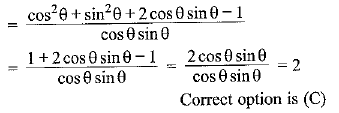(iii) (sec A + tan A) (1 - sin A)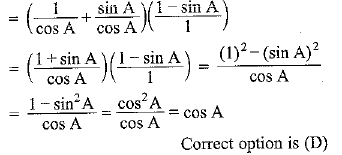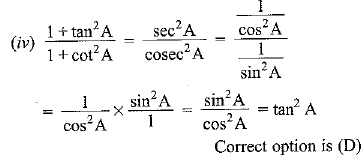Q.5. Prove the following identities, where the angles involved are acute angles for which the expressions are defined.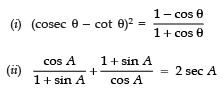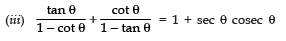[Hint: Write the expression in terms of sin θ and cos θ]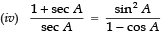[Hint: Simplify LHS and RHS separately]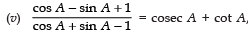using the identity cosec2 A = 1 + cot2 A.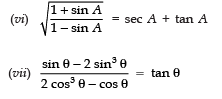(viii) (sin A + cosec A)2 + (cos A + sec A)2 = 7 + tan2 A + cot2 A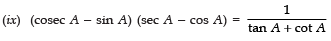[Hint: Simplify LHS and RHS separately]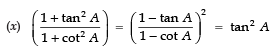Sol. (i) LHS = (cosec θ - cot θ)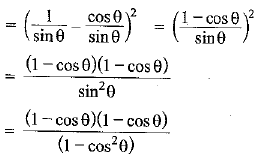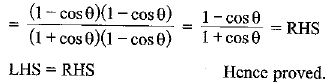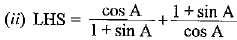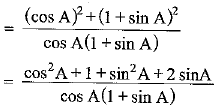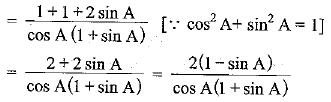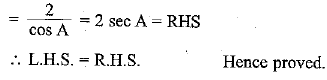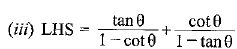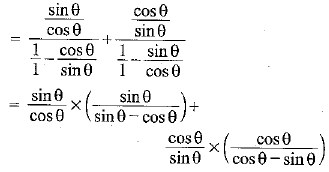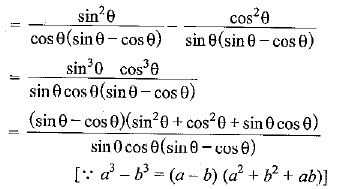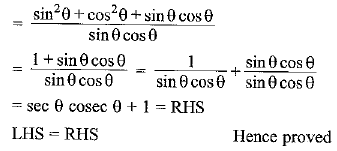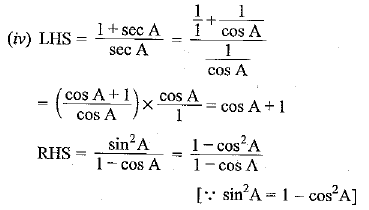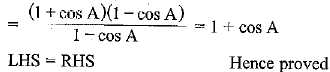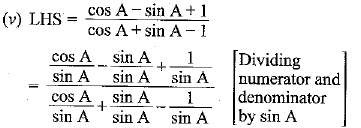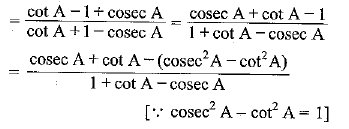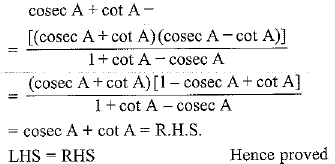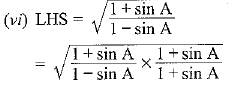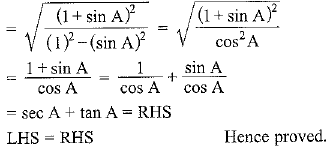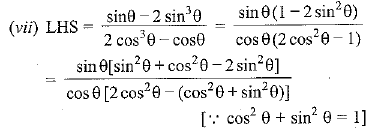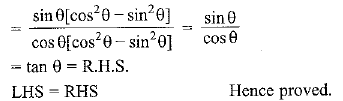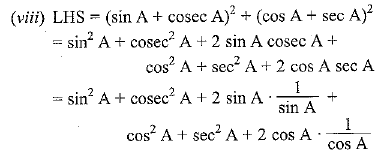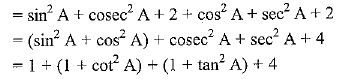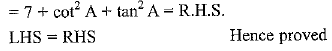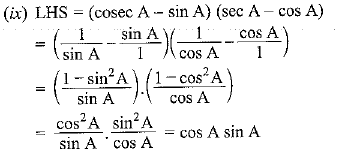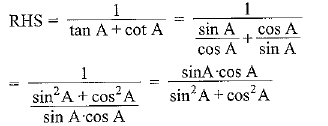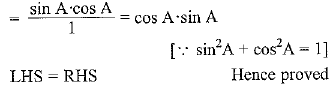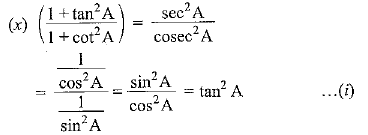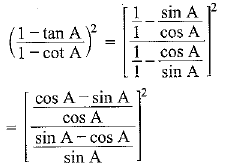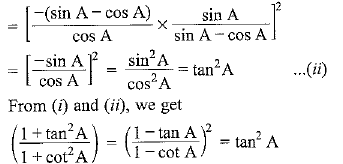Offer running on EduRev: Apply code STAYHOME200 to get INR 200 off on our premium plan EduRev Infinity!

## Mathematics (Maths) Class 10

178 videos|268 docs|103 tests

,

,

,

,

,

,

,

,

,

,

,

,

,

,

,

,

,

,

,

,

,

;